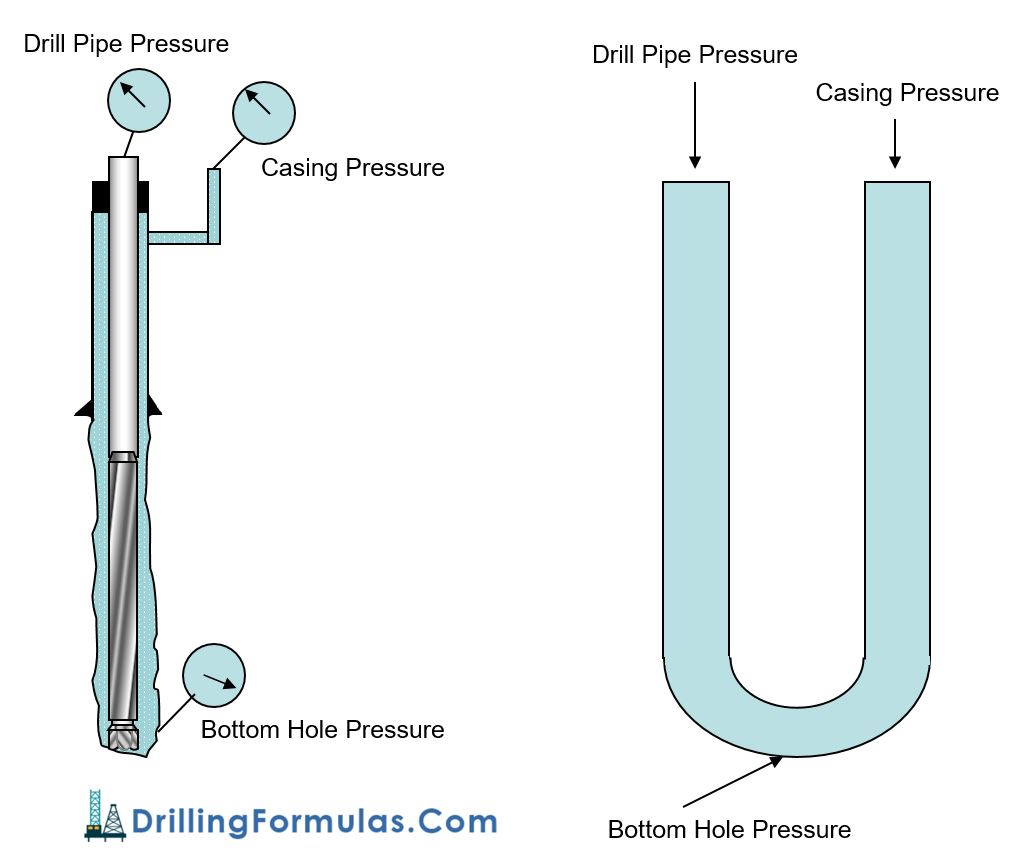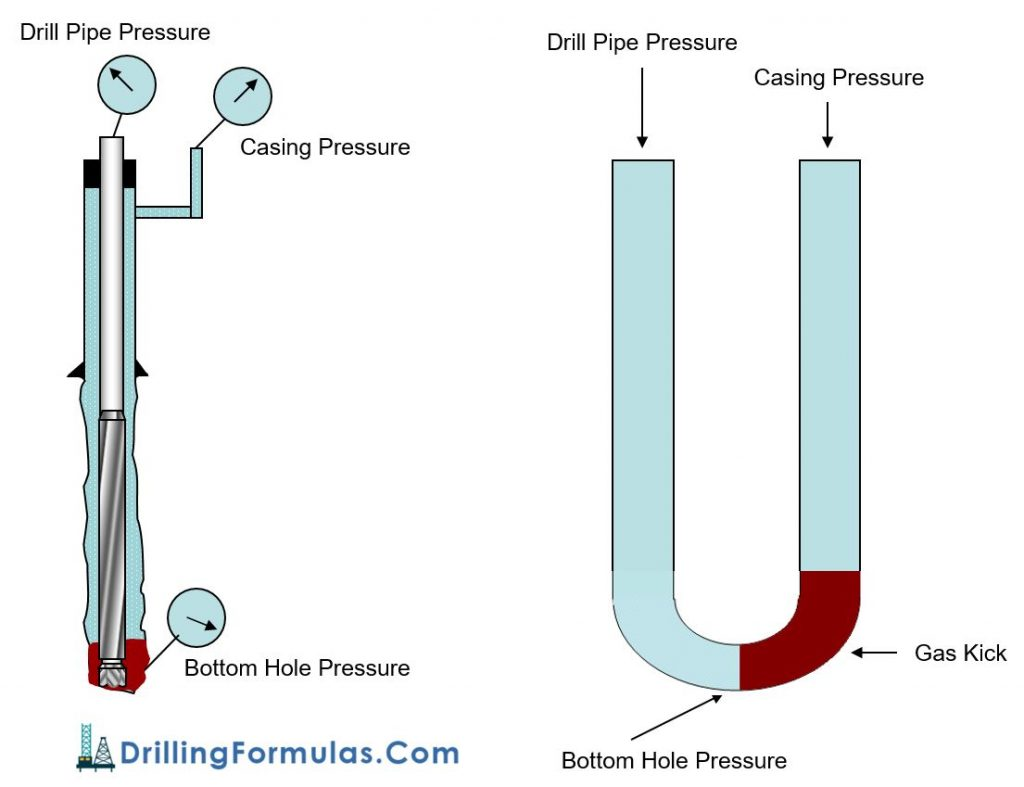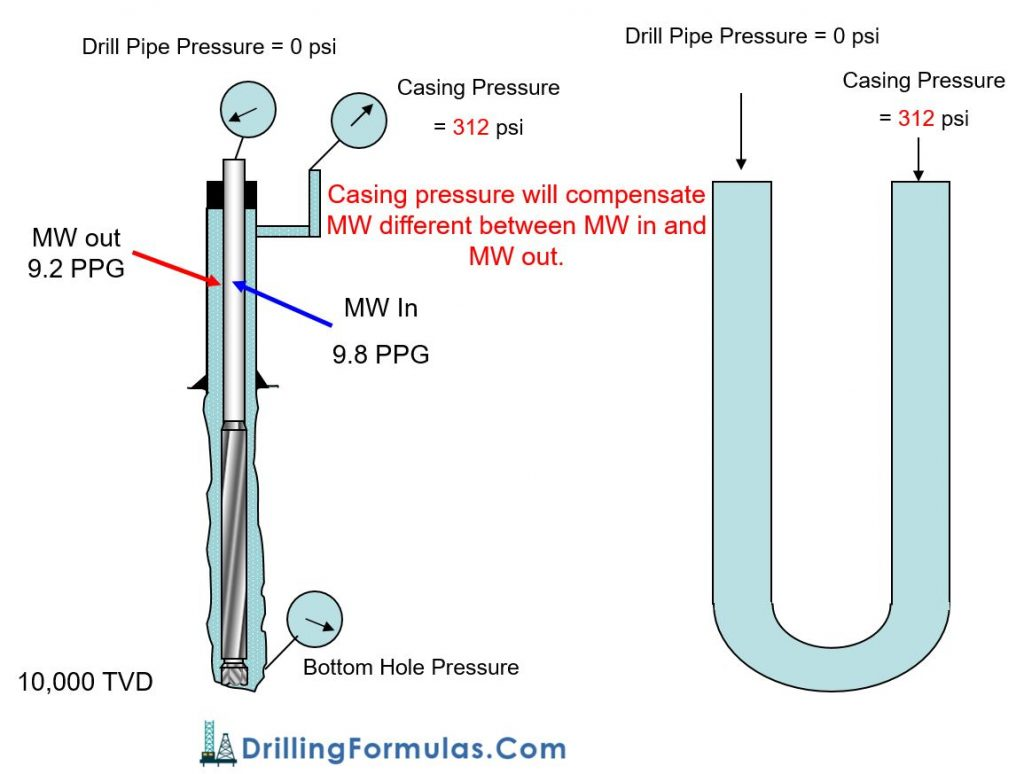# Understand U-Tube Concept and Importance of U-Tube

We can use the behavior of one of the fluid columns to describe behavior regarding what is happening in another side of fluid column, if two fluid columns are connected at bottom. Basically, this situation is simply described in common oil field name as “U Tube”.

In oil field especially drilling business, “U Tube” can be considered as a string of pipe (drill pipe and tubing) is in a wellbore and fluids are able to pass inside of string of pipe (drill pipe and tubing) and the annulus (area between wellbore and string of pipe). The figure 1 below demonstrates “U Tube” in our drilling business.Figure 1 – U-Tube Diagram Represents Both Sides of Fluid Columns

A horizontal tube connects the right-hand side of the U-Tube and fluid levels in both columns should equalize when a fluid with consistent density is added. Furthermore, the hydrostatic pressure should be equal at the bottom of both columns. The pressure found at the base of both columns is considered ‘bottomhole pressure’. To replicate the opening through the nozzles in the bit, the opening at the base exists.

The mathematical relationship for this is shown below;

## BHP = HP + SP

Where;

BHP = bottomhole pressure

HP = hydrostatic pressure

SP = surface pressure

With the U-tube concept applied, both sides of fluid columns can be described with the equation below;

## BHP = SIDPP + HP string = SICP + HP annulus

Where;

BHP = bottomhole pressure

SIDPP = shut in drillpipe pressure

HP string = hydrostatic pressure  in drill string

SICP = shut in casing pressure

HP annulus = hydrostatic pressure in annulus

When fluid density in both columns is equal, U-Tubes can be relatively simple. Surface pressures on the drillpipe and casing sides will be the same when the drillpipe and casing are themselves full of the same fluid density. However, U-Tubes become more difficult when fluids with varying densities are found in the columns. Despite the same BHP, both HP and SP will differ.

With hydrostatic and surface pressure equal in both columns, U-Tubes aren’t too interesting because both columns are filled with fluids of the same density. For example, when the annulus and drillpipe contain the same weight drilling mud while a bit is run to the hole’s bottom. The hydrostatic pressure is equal at both the casing and drillpipe side, fluid levels are static at the top, and the surface pressure on the drillpipe and casing sides are zero.

On the other hand, when columns are occupied by fluids of different densities, there’s likely to be a difference in both surface pressure and hydrostatic pressure in both columns (drillstring and casing side). For example, this is commonly seen in a kick with the bit on bottom as you can see from the figure 2 diagram. As formation pressure increases above hydrostatic pressure (generated by mud in the well), it kicks. The well will stop flowing if it’s shut-in; a surface pressure on the drillpipe gauge is then a reflection of the pressure underbalance. As opposed to drilling mud in the annulus, the fluid now contains lighter weight formation fluid and this leads to a reduction in total hydrostatic pressure (within the annulus). The shut-in casing pressure increases above shut-in drillpipe pressure to compensate to the underbalanced in the annulus side compared to the drillpipe side.Figure 2 – U-Tube Diagram Represents Both Sides of Fluid Columns with Gas Kick

Why is U-Tube very important?

It is very vital to keep a basic concept of U-Tube in mind.

If there are two different fluids between inside of string and annulus, fluids always flow from a higher pressure area to a lower pressure.

If the system is NOT closed, lighter fluid will be flown out and it will be stopped when system pressure is stabilized (see the figure 3 below).Figure 3 – U-Tube Diagram Represents Both Sides of Fluid Columns Without a Closed System

If the system is closed, for example the well shut in, pressure must be the same at the bottom point where both sides of U-tube are connected . Therefore, drill pipe pressure and casing pressure (annulus pressure) will be responded based on fluid in each side and formation pressure at bottom hole (see the figure 4 below).

Figure 4 demonstrates different in hydrostatic pressure between drill pipe and casing when mud weight 9.8 ppg is pumped to the bit and the well is shut in. This example helps understand how to use the equation to solve the problem.

The calculation is shown below.

## BHP = SIDPP + HP string = SICP + HP annulus

BHP = 0 + (0.052 × 10,000  × 9.8) = 5,096 psi

5,096 psi = SICP + (0.052 × 10,000  × 9.2)

5,096 psi =  SICP + 4,784 psi

SICP = 312 psiFigure 4 – U-Tube Diagram Represents Both Sides of Fluid Columns With a Closed System

The U-Tube concept can be widely applied in many drilling and workover application such as well control, cementing, hole monitoring, pulling out of hole, pumping slug, etc.

Ref books:

Lapeyrouse, N.J., 2002. Formulas and calculations for drilling, production and workover, Boston: Gulf Professional publishing.

Bourgoyne, A.J.T., Chenevert , M.E. & Millheim, K.K., 1986. SPE Textbook Series, Volume 2: Applied Drilling Engineering, Society of Petroleum Engineers.

Mitchell, R.F., Miska, S. & Aadny, B.S., 2011. Fundamentals of drilling engineering, Richardson, TX: Society of Petroleum Engineers.

Share the joy
Tagged , . Bookmark the permalink.1.Rowland Nwaobosi says: